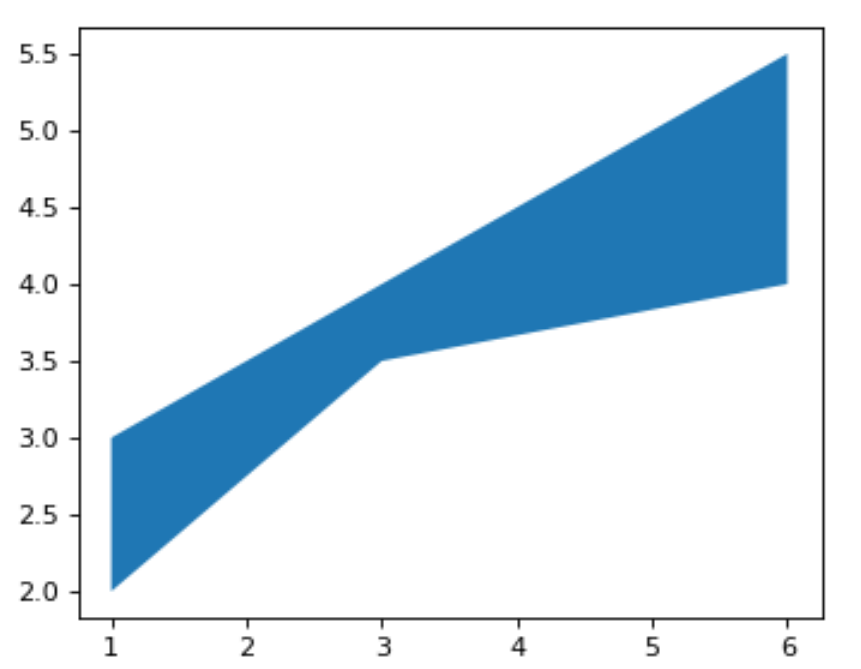# Make filled polygons between two curves in Python using Matplotlib

Matplotlib is an amazing visualization library in Python for 2D plots of arrays. Matplotlib is a multi-platform data visualization library built on NumPy arrays and designed to work with the broader SciPy stack.

To create filled polygons between two curves a PolyCollection filling needs to be created between y1 and y2.

1. x: It is an array of length N that holds data of x.
2. y1: It is an array or a scalar of length N that holds data of y.
3. y2:It is an array or a scalar of length N that holds data of y.

Example:

 `import` `matplotlib.pyplot as plt ` `import` `numpy as np ` `# set the width  ` `width ``=` `3.5` ` `  `# set the height ` `height ``=` `2.5` ` `  `# set the depth ` `depth ``=` `65` ` `  `# plot the figure ` `plt.figure(figsize ``=``(width, height), dpi ``=` `depth) ` ` `  `# set the x array of length 3 ` `x ``=` `[``1``, ``3``, ``6``] ` ` `  `# set y1 array of length 3 ` `y1 ``=` `[``2``, ``3.5``, ``4``] ` ` `  `# set y2 array of length 3 ` `y2 ``=` `[``3``, ``4``, ``5.5``] ` ` `  `# fill the horizontal arean between y1 and y2 ` `plt.fill_between(x, y1, y2) ` ` `  `# show the plotted figure ` `plt.show() `

Output:My Personal Notes arrow_drop_upCheck out this Author's contributed articles.

If you like GeeksforGeeks and would like to contribute, you can also write an article using contribute.geeksforgeeks.org or mail your article to contribute@geeksforgeeks.org. See your article appearing on the GeeksforGeeks main page and help other Geeks.

Please Improve this article if you find anything incorrect by clicking on the "Improve Article" button below.

Article Tags :

Be the First to upvote.

Please write to us at contribute@geeksforgeeks.org to report any issue with the above content.